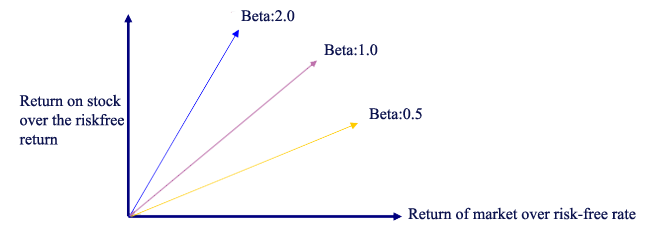Shopping Cart

# Beta Coefficient

#### The beta coefficient is a measure of the volatility of an individual stock in comparison to the volatility of the entire market. Beta coefficient calculates the expected return on an asset considering systematic risk of its volatility in comparison to the market’s return of investment and its volatility/risk. The beta formula is used in the Capital Asset Pricing Model (CAPM) for calculating the rate of return of a stock or portfolio and calculate the Cost of Equity as shown below: Cost of Equity = Risk Free Rate + Beta x Risk Premium